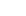﻿
﻿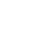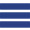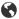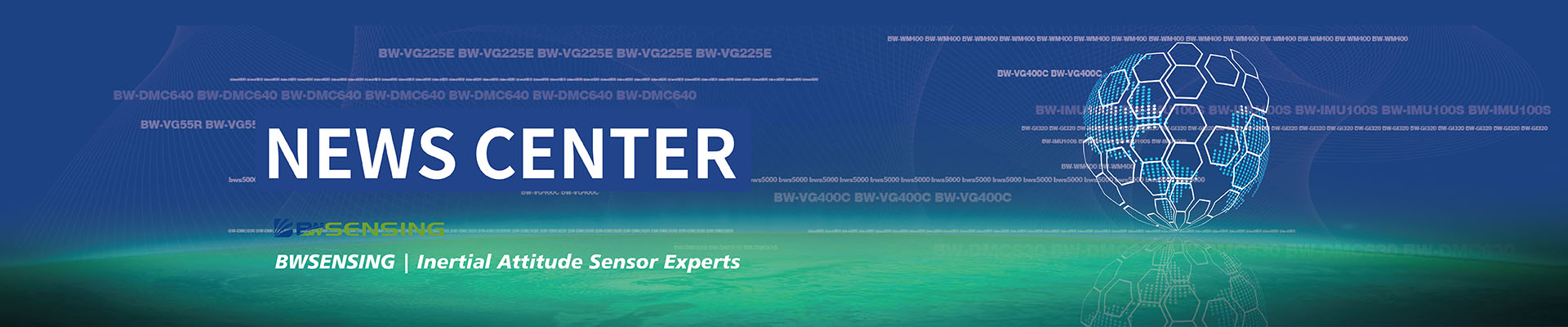All kinds of tilt sensors can be categorized in this way Release date：2017-09-27   Browsing volume：2510

Horizontal distance and the height of the object of the whole system can use the obliquity sensor, based on the basic principle of tilting Angle sensor can be divided into solid pendulum, liquid, gas pendulum such three kinds of Angle sensor, in the final analysis they are using the earth's gravity, express sensors in analog signal or the pulse signal of the attitude Angle of the earth, namely with the earth gravity Angle (tilting Angle) the physical quantities. It's a creative transformation.

Solid pendulum tilt sensor

Solid mostly adopt the force balance in the design of the servo system, it is composed of pendulum, cycloid, stents, pendulum is pulling force by gravity G and T, the force F = Gsin Ɵ = mgsine Ɵ, type of theta Angle for cycloid and vertical direction. When measured in a small Angle, it can be considered that F is linearly dependent with theta. For example, our common strain dip sensor is based on this principle.

Liquid pendulum tilt sensor

The structural principle of the liquid pendulum Angle sensor is to have the conductive fluid inside the glass shell, and there are three platinum electrodes and the external phase connection. The three electrodes are parallel and equal to each other, as shown in FIG. 2. When the shell is horizontal, the electrode is inserted into the same depth. If equal between the two electrodes and amplitude of voltage, will form ionic current between the electrode, resistance of liquid between two electrodes is equivalent to two RI3, as shown in the left electrode immersed depth is small, the conductive liquid, decrease the number of ion conductive, resistance RI decreases, namely RI > RIII. Conversely, RI < RIII if it tilts in the opposite direction. Increase in the relative pole, the conductivity increases, the conductivity increases, and the resistance RIII and RIII. RI = RIII if the liquid is at the level of the pendulum. When the glass housing is tilted, the conductive fluid between the electrodes is not equal, and the depth of the three electrodes immersed in the liquid also changes, but the intermediate electrode immersion depth remains basically unchanged.

Gas pendulum tilt sensor

Gas when heated by the effect of buoyancy lift, like a pendulum and solid place also has the sensitive as much as quality of liquid, the heat flow is always trying to keep in the vertical direction, therefore also has the characteristics of the pendulum. The gas pendulum Angle sensor consists of a closed cavity, a gas and a hot line. When the relative horizontal tilt the plane cavity or chamber under the effect of acceleration, the hotline resistance changes, and hotline resistance changes is a function of Angle q or acceleration, and therefore also has the effect of the pendulum. The change of the hot-wire resistance is caused by the exchange of energy between the gas and the hot line. The sensitive mechanism of "gas pendulum" type inertial device is based on energy transfer in the airtight cavity. There is a gas and hot line in the closed cavity, and the hot line is the only heat source. When the device is energized, the gas is heated. Convection is the main form in the heat transfer of the hot line.

Under the influence of gravity field, the sensitive mass of liquid pendulum is electrolyte, and the sensitive mass of the pendulum is the quality of pendulum, while the sensitive mass of gas pendulum is gas. The gas is the only body in the chamber, and its mass is small. Therefore, the inertial force produced when the shock or high overload is small, has a strong vibration or impact energy. But we all know that the gas movement control is very complicated, which affects its movement more, its precision cannot meet the requirement of military weapon system. The solid pendulum Angle sensor has a clear pendulum length and a pendulum, and its mechanism is basically the same as the acceleration sensor.

The dip Angle sensor has many kinds of products in practical use, such as electromagnetic pendulum, and its product measuring range, precision and anti-overload ability are high, and its application is widely used in weapon system. The liquid pendulum Angle sensor is between the solid state and the gas phase, which combines the advantages and disadvantages of the gas solid. It has the characteristic of system stability, in the high precision system, the application is more extensive, and the domestic and foreign products are more of this kind.

• STAY CONNECTED
• 24 Hours Service Online

WhatsApp/Tel：+86 15050 672 146

+86 17606 118 008

Commercial：sales@bwsensing.com

Customer Service：support@bwsensing.com

••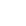+86 189 2129 2620
+86 176 0611 8008
•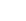sales@bwsensing.com
•